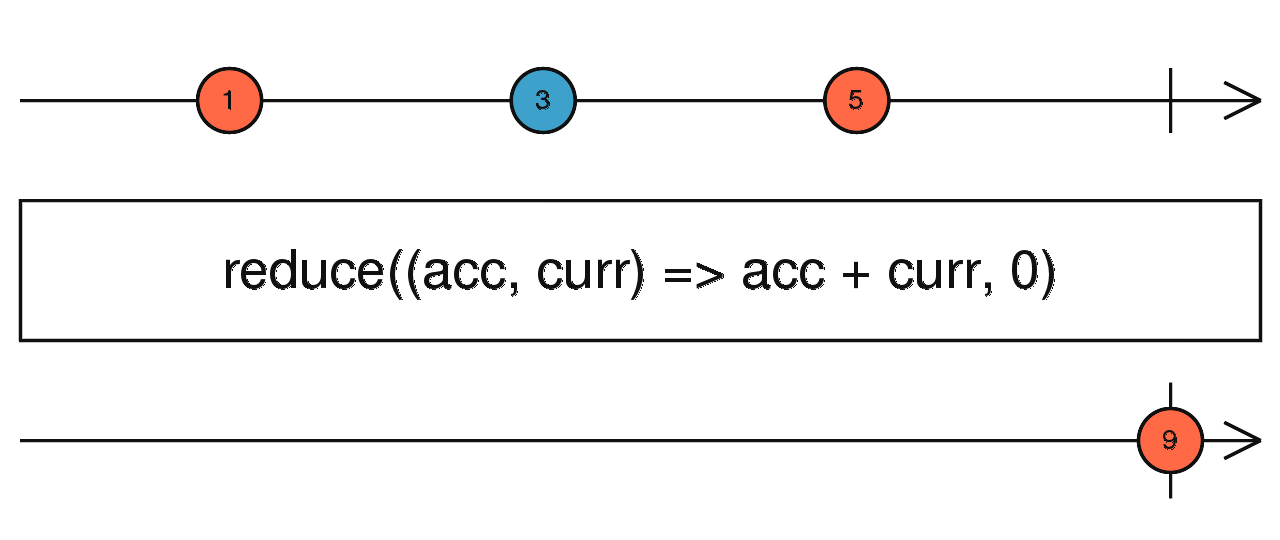/RxJS

# reduce

`function` `stable`

Applies an accumulator function over the source Observable, and returns the accumulated result when the source completes, given an optional seed value.

### `reduce<T, R>(accumulator: (acc: T | R, value: T, index?: number) => T | R, seed?: T | R): OperatorFunction<T, T | R>`

#### Parameters

 accumulator The accumulator function called on each source value. seed Optional. Default is `undefined`. The initial accumulation value.

#### Returns

`OperatorFunction<T, T | R>`: An Observable that emits a single value that is the result of accumulating the values emitted by the source Observable.

## Description

Combines together all values emitted on the source, using an accumulator function that knows how to join a new source value into the accumulation from the past.Like Array.prototype.reduce(), `reduce` applies an `accumulator` function against an accumulation and each value of the source Observable (from the past) to reduce it to a single value, emitted on the output Observable. Note that `reduce` will only emit one value, only when the source Observable completes. It is equivalent to applying operator `scan` followed by operator `last`.

Returns an Observable that applies a specified `accumulator` function to each item emitted by the source Observable. If a `seed` value is specified, then that value will be used as the initial value for the accumulator. If no seed value is specified, the first item of the source is used as the seed.

## Example

Count the number of click events that happened in 5 seconds

```import { fromEvent, interval } from 'rxjs';
import { reduce, takeUntil, mapTo } from 'rxjs/operators';

const clicksInFiveSeconds = fromEvent(document, 'click').pipe(
takeUntil(interval(5000)),
);
const ones = clicksInFiveSeconds.pipe(mapTo(1));
const seed = 0;
const count = ones.pipe(reduce((acc, one) => acc + one, seed));
count.subscribe(x => console.log(x));```

### `reduce(accumulator: (acc: R, value: T, index: number) => R, seed: R): OperatorFunction<T, R>`

#### Parameters

 accumulator Type: `(acc: R, value: T, index: number) => R`. seed Type: `R`.

#### Returns

`OperatorFunction<T, R>`

### `reduce(accumulator: (acc: T, value: T, index: number) => T, seed?: T): MonoTypeOperatorFunction<T>`

#### Parameters

 accumulator Type: `(acc: T, value: T, index: number) => T`. seed Optional. Default is `undefined`. Type: `T`.

#### Returns

`MonoTypeOperatorFunction<T>`

### `reduce(accumulator: (acc: R, value: T, index: number) => R): OperatorFunction<T, R>`

#### Parameters

 accumulator Type: `(acc: R, value: T, index: number) => R`.

#### Returns

`OperatorFunction<T, R>`